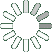Puzzle Command
Daily Puzzle
Number Logic #5
December 13, 2014Find a 5 digit number where:

The 1st digit is equal to 9.
The 2nd digit is the number of 2s in the number.
The 3rd digit is the number of 3s in the number.
The 4th digit is the number of 9s in the number.
The 5th digit is any number.

The digits in the number compose a palindrome.

Puzzle Notes
5 digit numbers range from 10,000 to 99,999.
The digits in the number compose a palindrome.
Enter in your solution below and click submit.